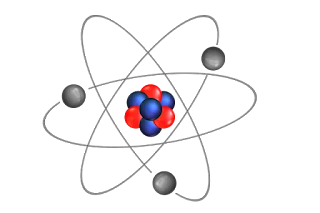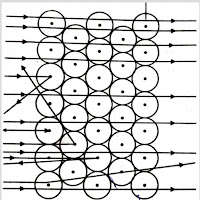# what is the structure of atoms ?

###An atom is an smallest structure of an element, which posses electrons(negatively charged ion), proton(positively charged ion) & nucleus in the center of the atom.

Thomson's model of an atom :jj thomson model of the atom
J.J Thomson was the first man to propose a model for the structure of an atom.He proposed a spherical shape of an atom in which the electrons are embedded  in it, which is quite similar to the Christmas pudding.

### Thomson atomic theory : Thomson atomic theory proposed that :

i) An  atom consists of a positively charged sphere and the electrons are embedded in it.

ii)The negative and positive charges are equal in magnitude. So the atom as a whole is electrically neutral.

### Thomson model of atom drawbacks:

According to Thomson, atoms are electrically neutral in nature, whereas the experimental results carried out by other scientists could not explained this model.

### Rutherford model of atom class 9

After the failure of Thomson, in order to explain the structure of an atom,  a scientist  E.Rutherford attempted to explain the structure of an atom.The  Result of the experiment is that" most of the fast moving alpha particles passed straight through the gold foil,  whereas some were deflected by the foil by small angles and surprisingly one out of every 12000 particles appeared to rebound".

Based on this experiment, Rutherford put forward the nuclear model of an atom, which have the following characteristics:

i)There is a positively charged center in an atom known as nucleus. Nearly all the mass of an atom resides in the nucleus.

ii) The electron(negatively charged ion) moves around the nucleus in circular path.

iii) Lastly he said that the size of the atom is very minute as compared to the size of the size of the atom.

### Drawbacks of Rutherford model of an atom:-

The stability of electron in an orbit has become an major issue.Any particle in a circular orbit would undergo acceleration and during acceleration that particular charged particle would radiate energy, which leads the revolving electron would lose energy and finally falls into the nucleus.If this were so, the atom would  be highly unstable and hence matter would  not exist that we came to know, as the atoms are quite stable.

### Bohr's model of the atom :bohr model of atom

After the failure of  Rutherford Model, Neil Bohr successfully proved  the structure of an atom.He got Nobel prize for this achievement in 1922.

According to Bohr, an atom consists of a nucleus and around it the electrons revolves in the discrete orbits or shell which he named that energy levels as shown in above figure.The energy levels are represented by the letters K,L,M,N... or by the numbers n= 1,2,3,4....

### postulates of Bohr model of atom  :

The following Bohr's Postulates for describing the structure of an  atom is given by:

i)Only certain special orbits are allowed inside the atom, which are known as discrete orbits of electron.

ii) The electrons do not radiate energy while revolving in discrete orbits

### Neutrons   &  who discovered neutrons :

A scientist named J.Chadwick discovered neutrons.According to him, neutrons is a sub-atomic particles which had no charge(neutral particle) & a mass nearly identical to proton.

The new sub-atomic particle neutron is present in all atoms except hydrogen & it is represented by the symbol "n".

This new invention gives rise to another formula of  mass of an atom, which is given by:

Mass of atom = (mass of  proton + sum of neutrons) present in nucleus

### Atomic number  or  atomic number definition

An atomic number  is defined as the number of proton present in the nucleus  of an atom.

It is  represented by the symbol "Z".

### atomic number 1 to 30 :

1) Atomic number of hydrogen =1

2)  Atomic number of helium =2

3)Atomic number of lithium  =3

4)Atomic number of Beryllium=4

5)Atomic number of Boron=5

6)Atomic number of Carbon=6

7)Atomic number of Nitrogen=7

8)Atomic number of oxygen=8

9)Atomic number of fluorine=9

10)Atomic number of Neon=10

11)Atomic number of Sodium =11

12)Atomic number of Magnesium=12

13)Atomic number of Aluminium =13

14)Atomic number of Silicon =14

15)Atomic number of Phosphorus=15

16)Atomic number of Sulphur =16

17)Atomic number of Chlorine =17

18)Atomic number of Argon=18

19)Atomic number of Potassium =19

20)Atomic number of Calcium =20

21)Atomic number of Scandium =21

22)Atomic number of Titanium =22

24)Atomic number of Chromium =24

25)Atomic number of Manganese=25

26)Atomic number of Iron=26

27)Atomic number of Cobalt=27

28)Atomic number of Nickel  =28

29)Atomic number of Copper =29

30)Atomic number of Zinc =30

### Atomic mass of elements

From the properties of  sub-atomic particles of an atom, that the mass of an atom is  only due to the mass of proton and neutrons alone. As we know that this are present in the nucleus of an atom.Hence we can conclude that the mass of an atom.

Lets take an example to make it easier for understand:

e.g.The atomic mass of carbon is 12u, since it has 6protons and 6 neutrons.

### Atomic mass definition

An atomic mass of an element is defined as the sum of total number of protons and neutrons present in the nucleus of any atom.periodic table with atomic mass

### Isotopes :

It has  been found that a number of elements exists in nature which have the same atomic number  but different mass number and that particular elements are named as isotopes.i.e.isotopes of an element have atomic number and varied mass number.

### isotopes with example :

Isotopes for hydrogen- Protium, deutrium and tritium

Isotopes of carbon-Chlorine

All  of the above   are the isotopes of each other which posses same atomic number but varied mass number.

### Isotopes application :

i)Isotopes of cobalt is used in the treatment of cancer.

ii)Isotopes of iodine is used in then treatment of goitre

which isotopes of hydrogen is radioactive ?

Ans:- Isotopes of uranium.

### Isobars  :

Like isotopes a number of elements exists in nature which have the same mass number, but varied atomic number  and those elements are known as isobars.

### Isobars examples  :

Argon and calcium have atomic number 18 and 20 respectively but those same atomic mass 40i.e. they both posses same total number of protons and neutrons in each atom.

(Thank You for visiting our post..if you guyss have any doubt about the topic comment on the comment section and also visit our facebook page and instagram page.For more upcoming post click the bell icon to get notified .)